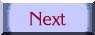Selected Abstracts

== Acrylic on Canvas ==

by Kathy Cunningham

2019

Oblong shapes in various sizes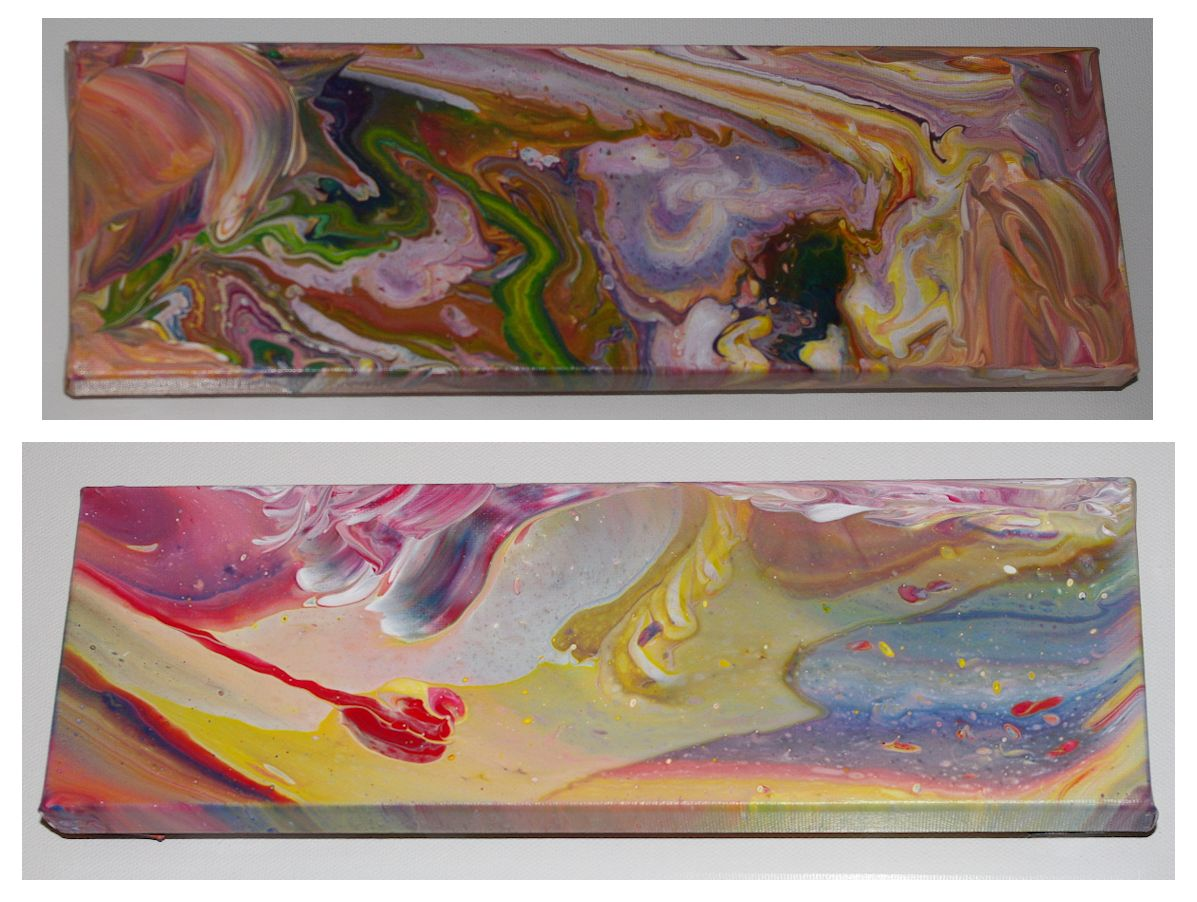Each is 4" x 12"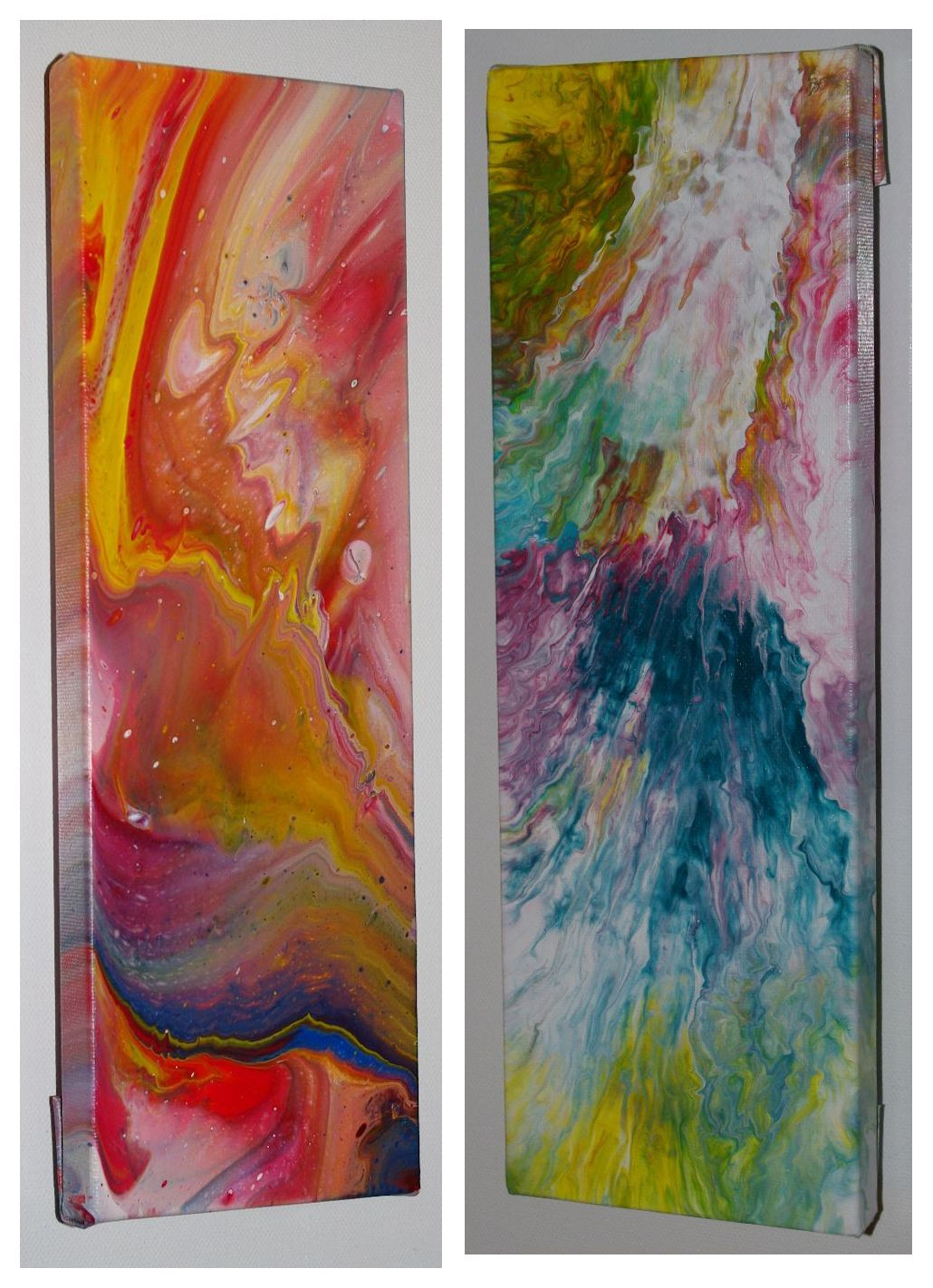Left is 12" x 4";  Right is 9" x 3"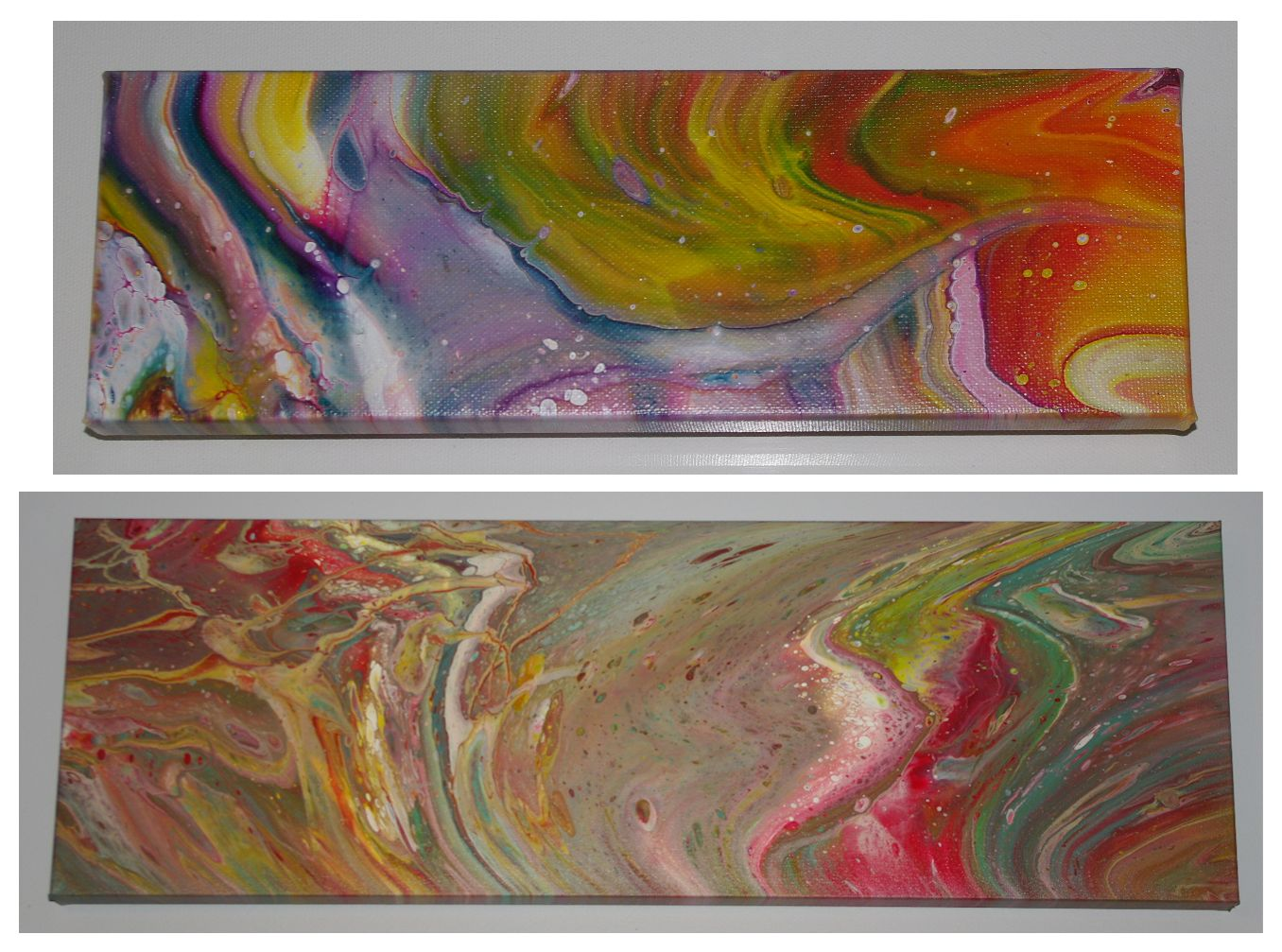Top is 4" x 12'" ; Bottom is 12" x 36"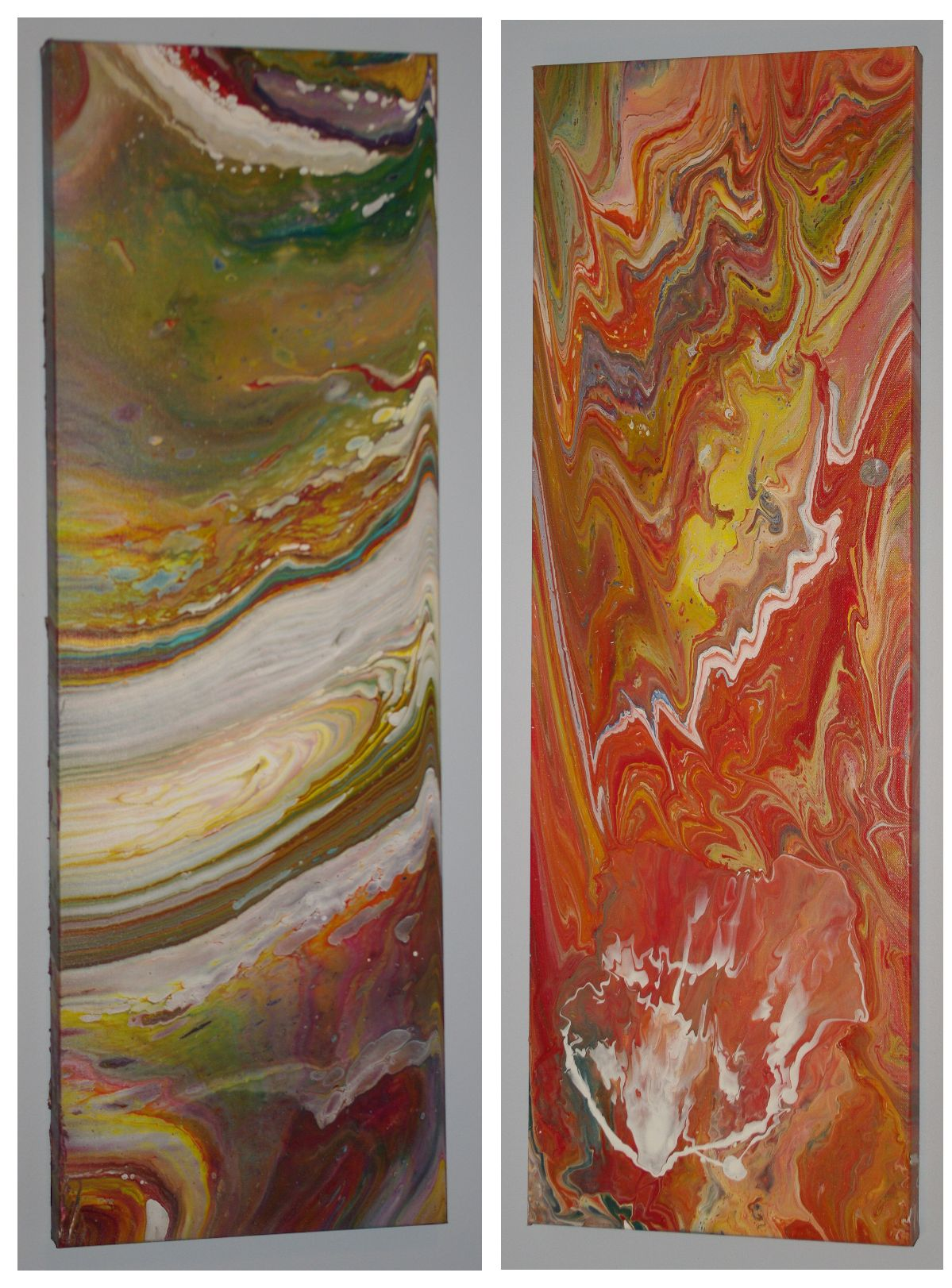Each is 36" x 12"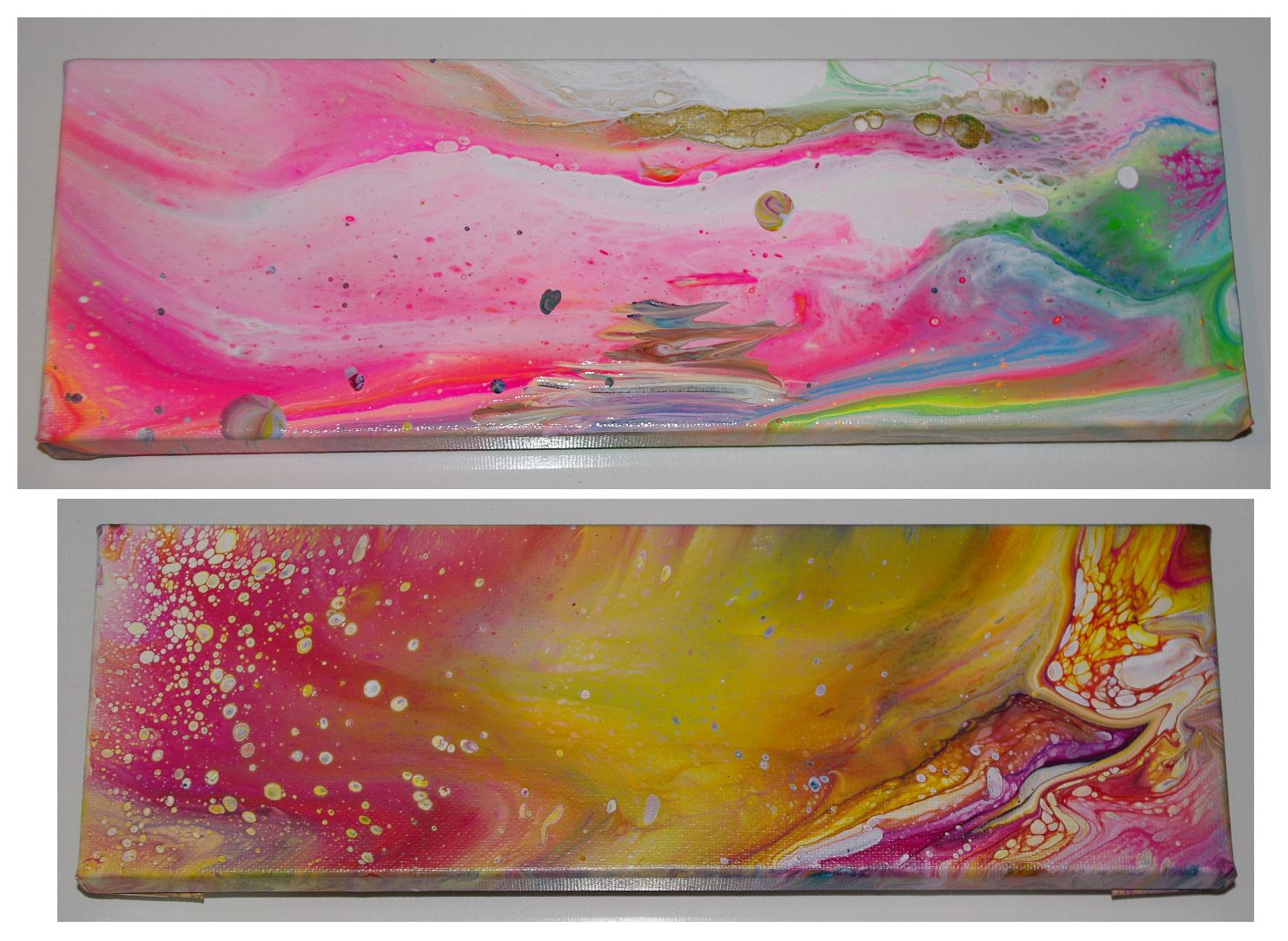Each is  4" x 12"

Next are several oblongs,
each is 9" x 3" (or 3" x 9")!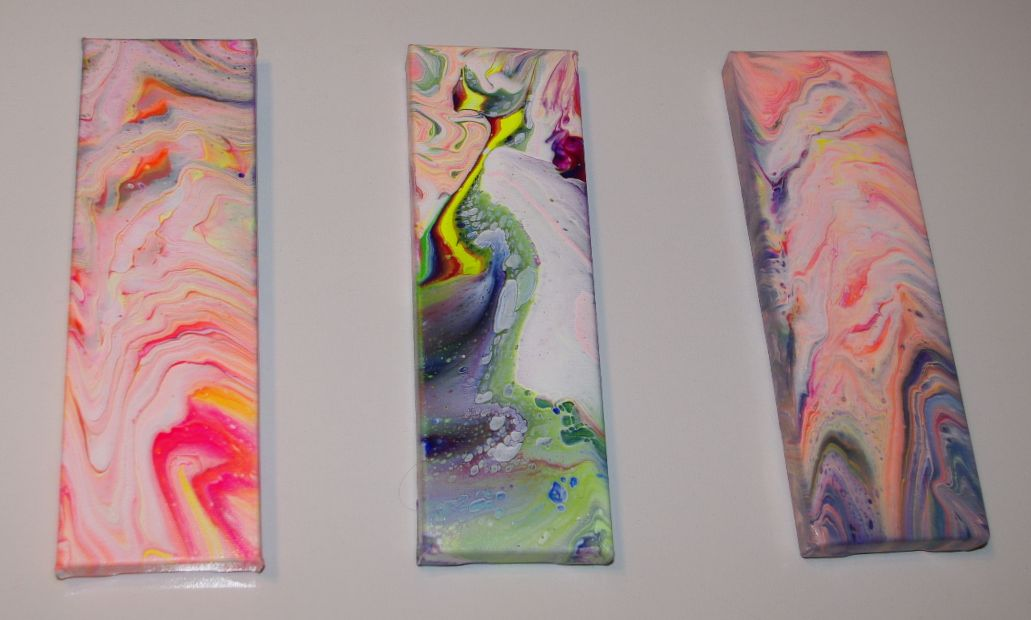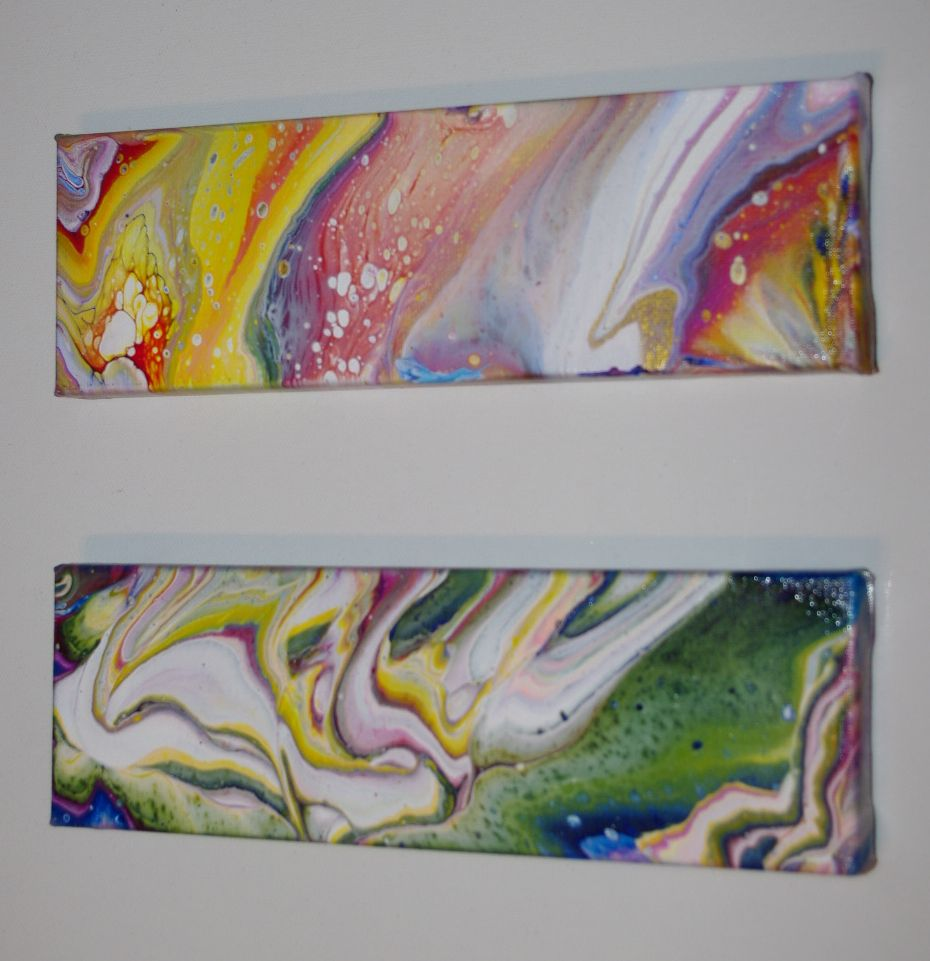Next are several other shapes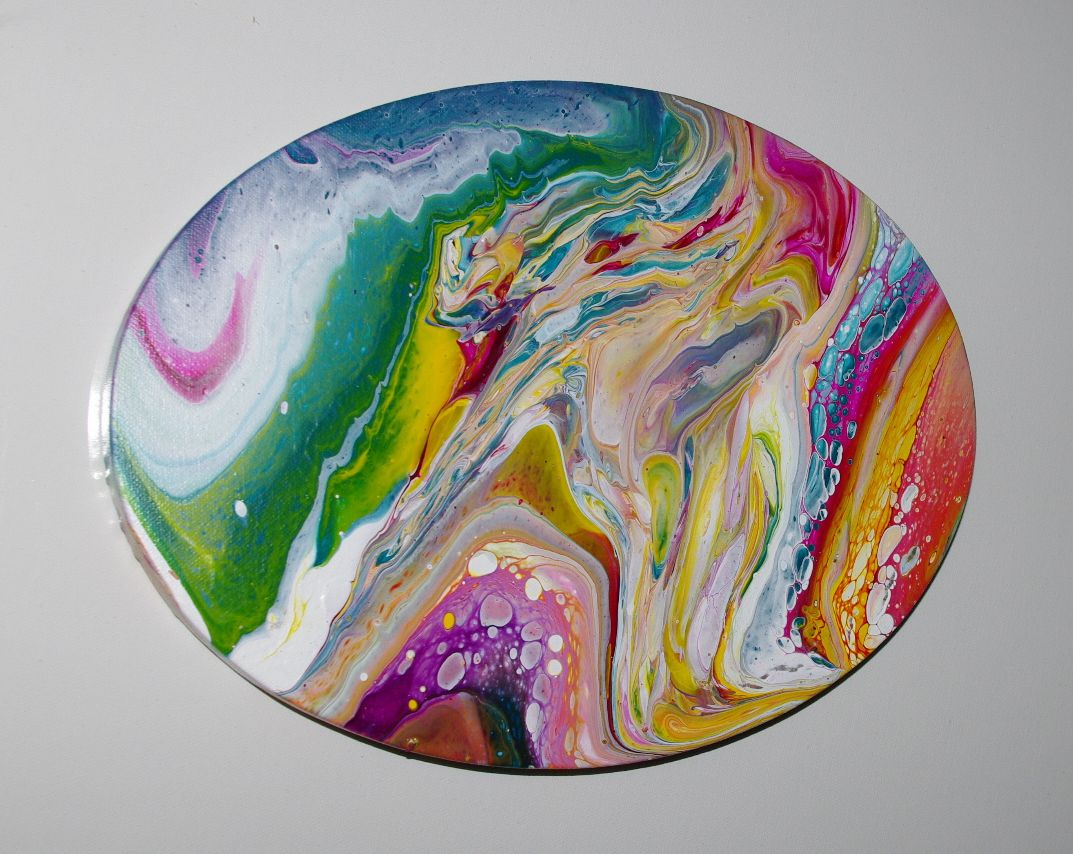Oval is 9" x 12"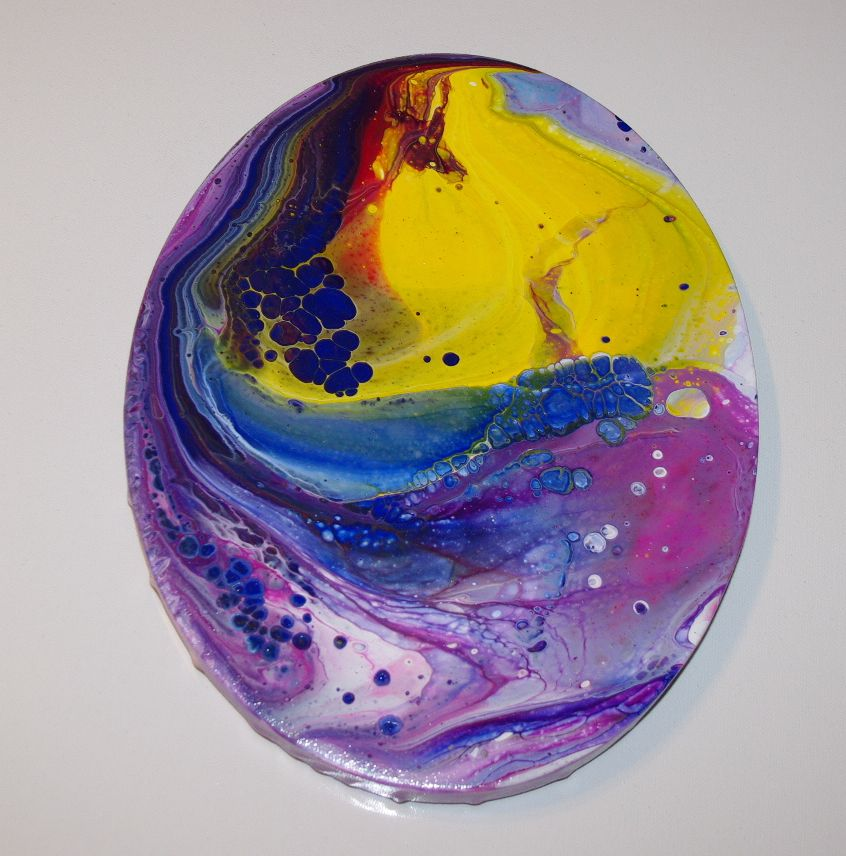Oval is 10" x 8"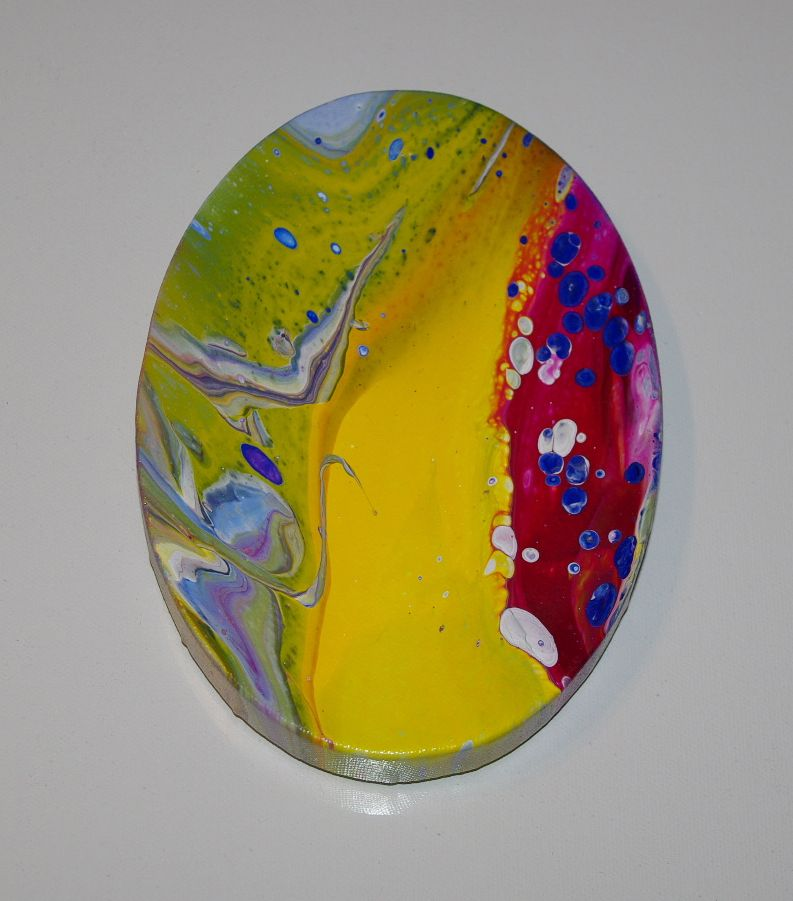Oval is 9" x 6"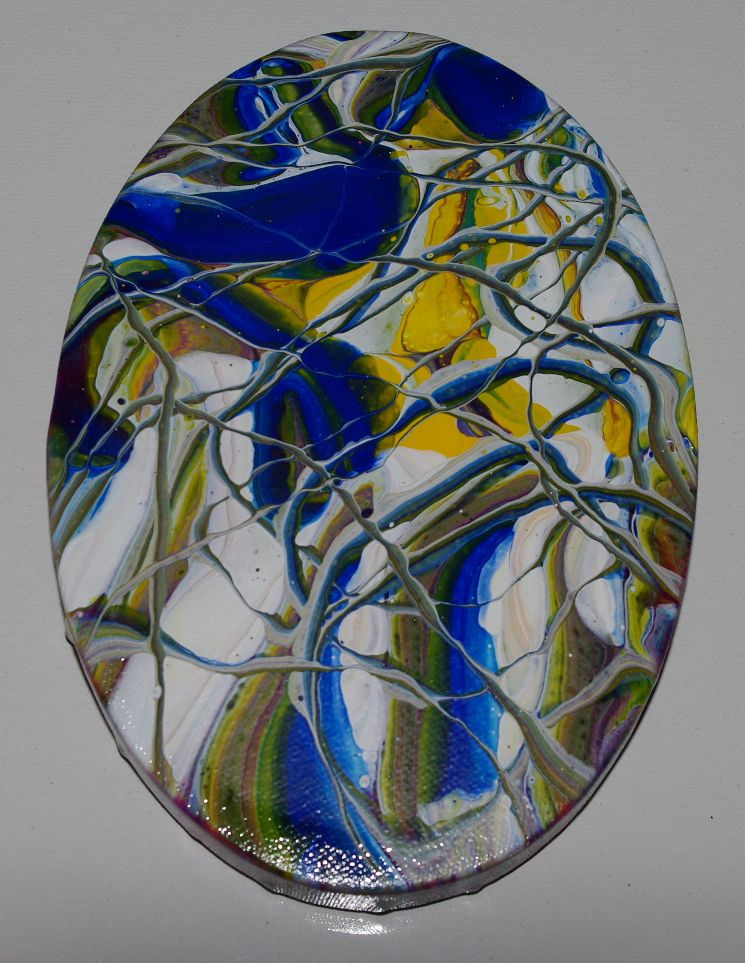Oval is 7" x 5"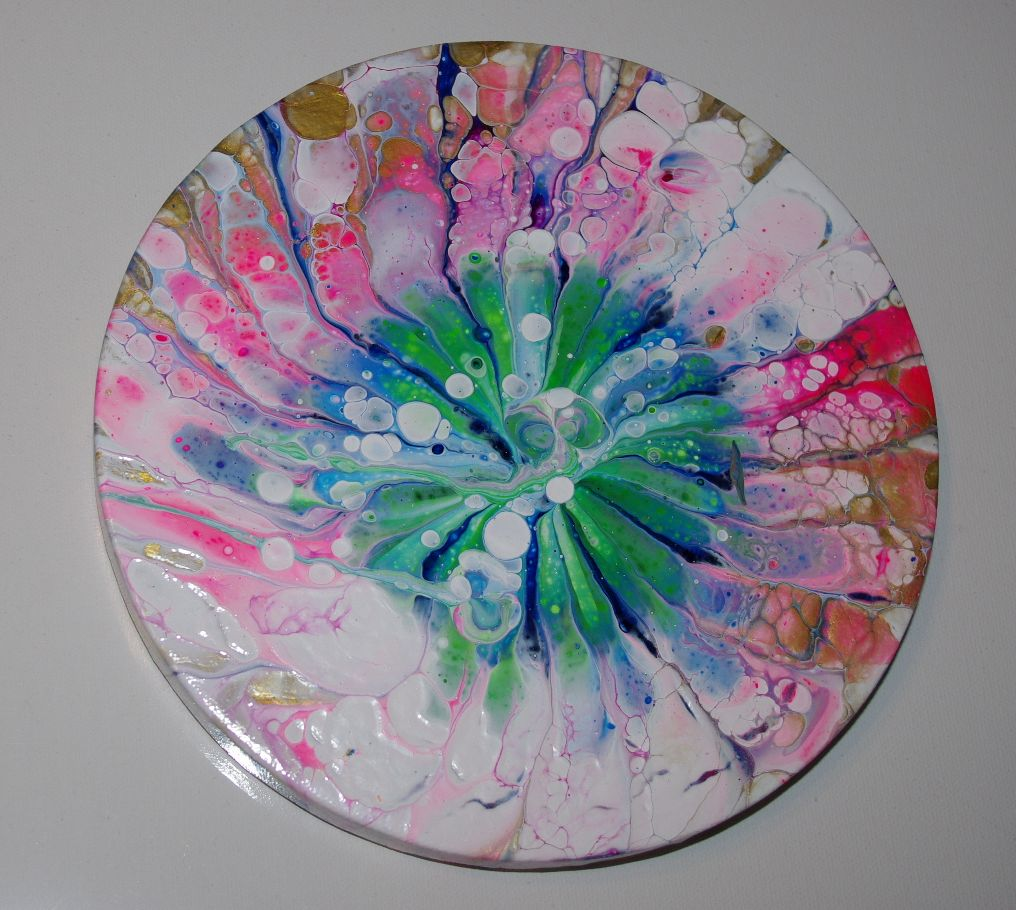Circle is 8" diameter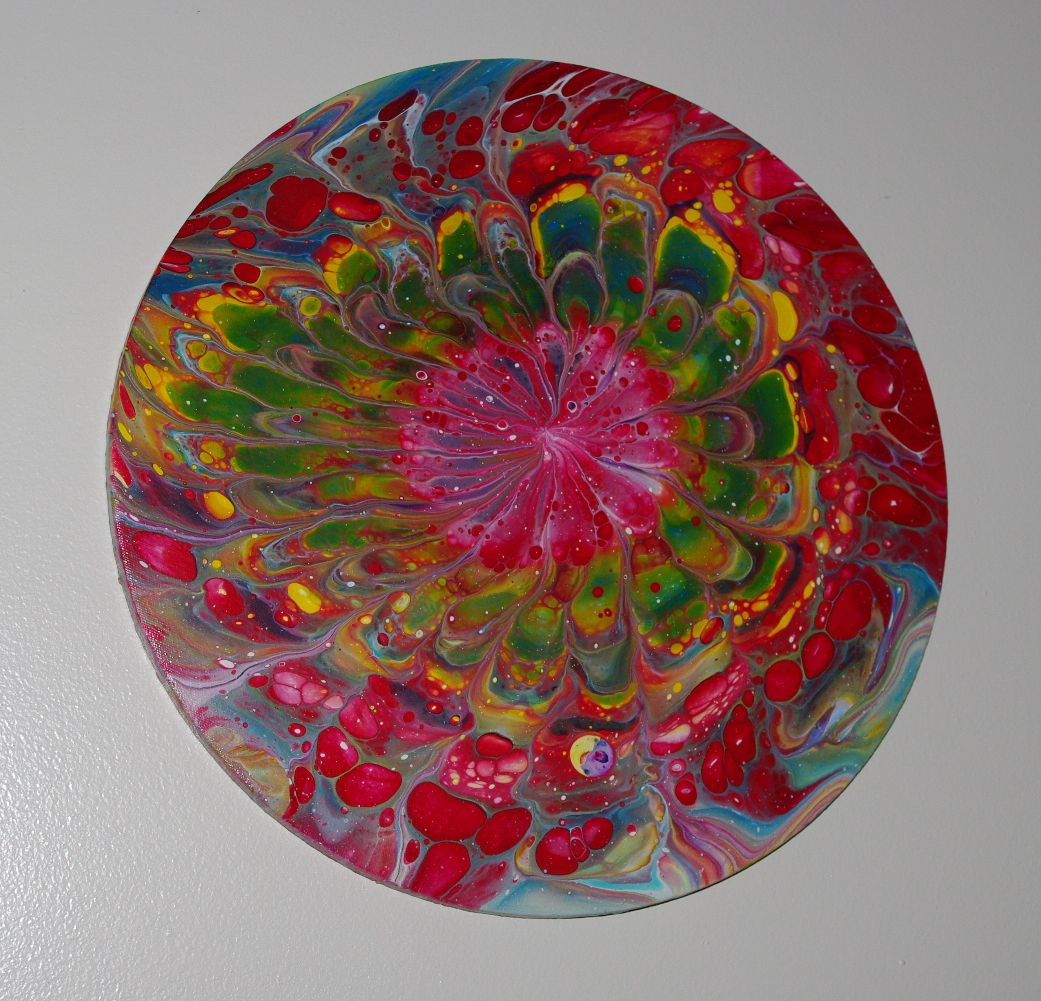Circle is 16" diameter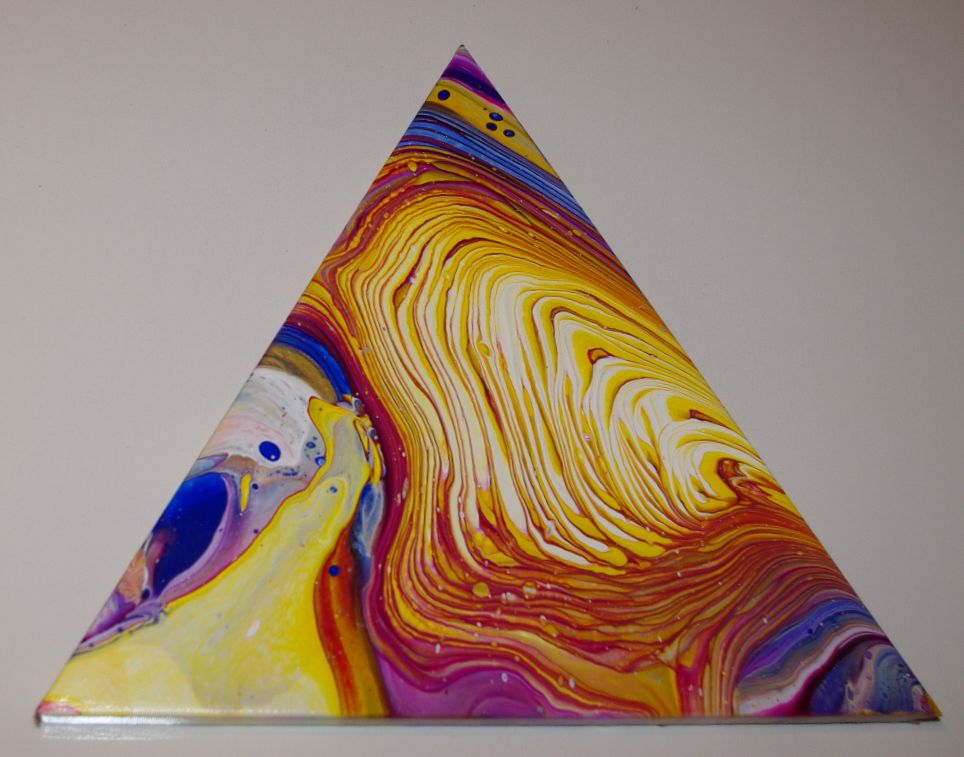Triangle is 14" x 11"Triangle is 7.5" x 6.5"Hexagon is 10" x 11.5"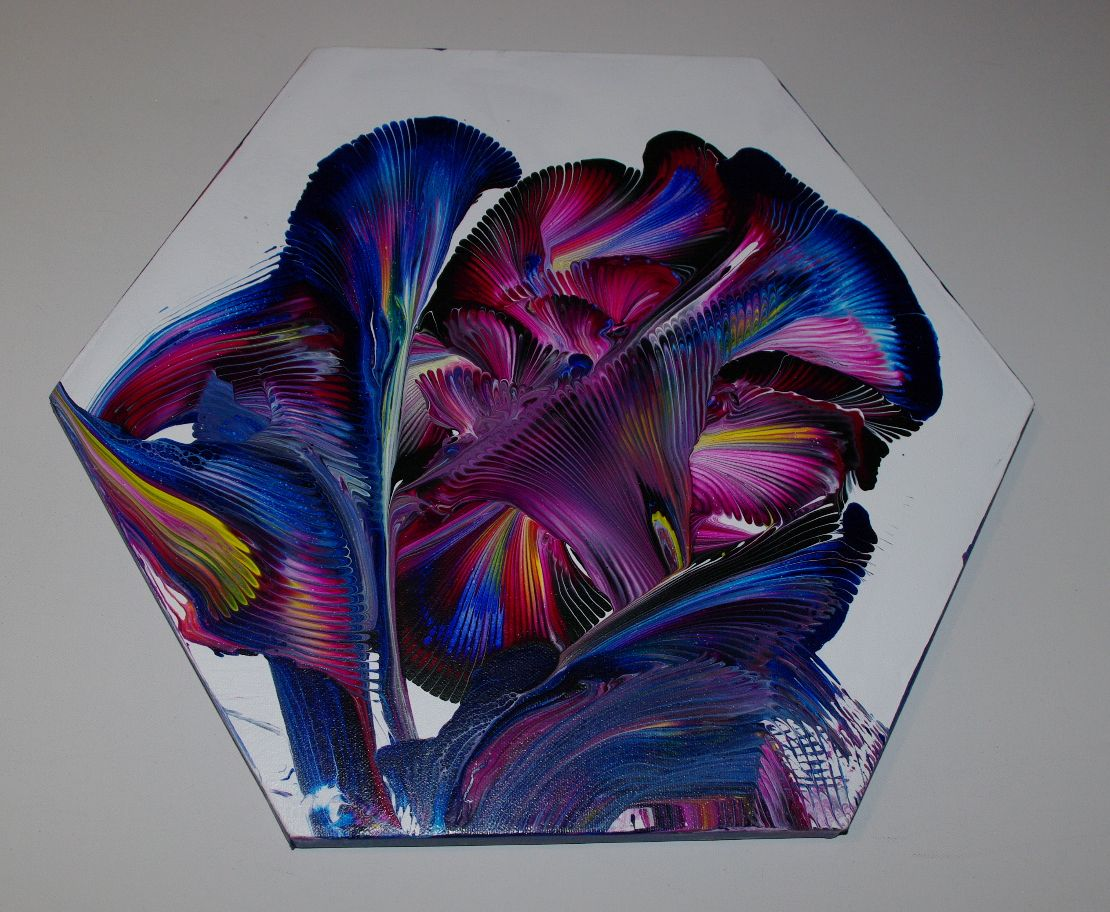Hexagon is 14" x 16"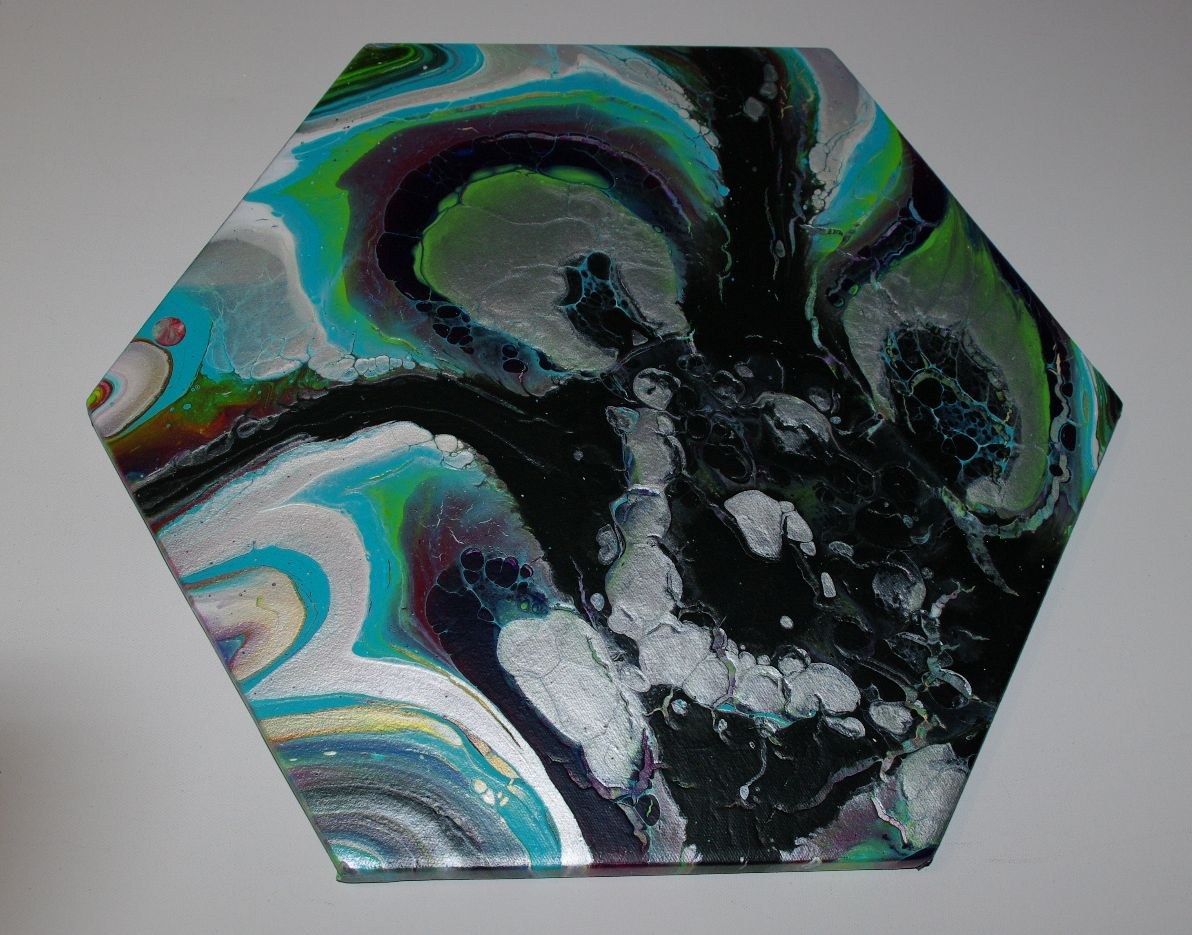Hexagon is 14" x 16"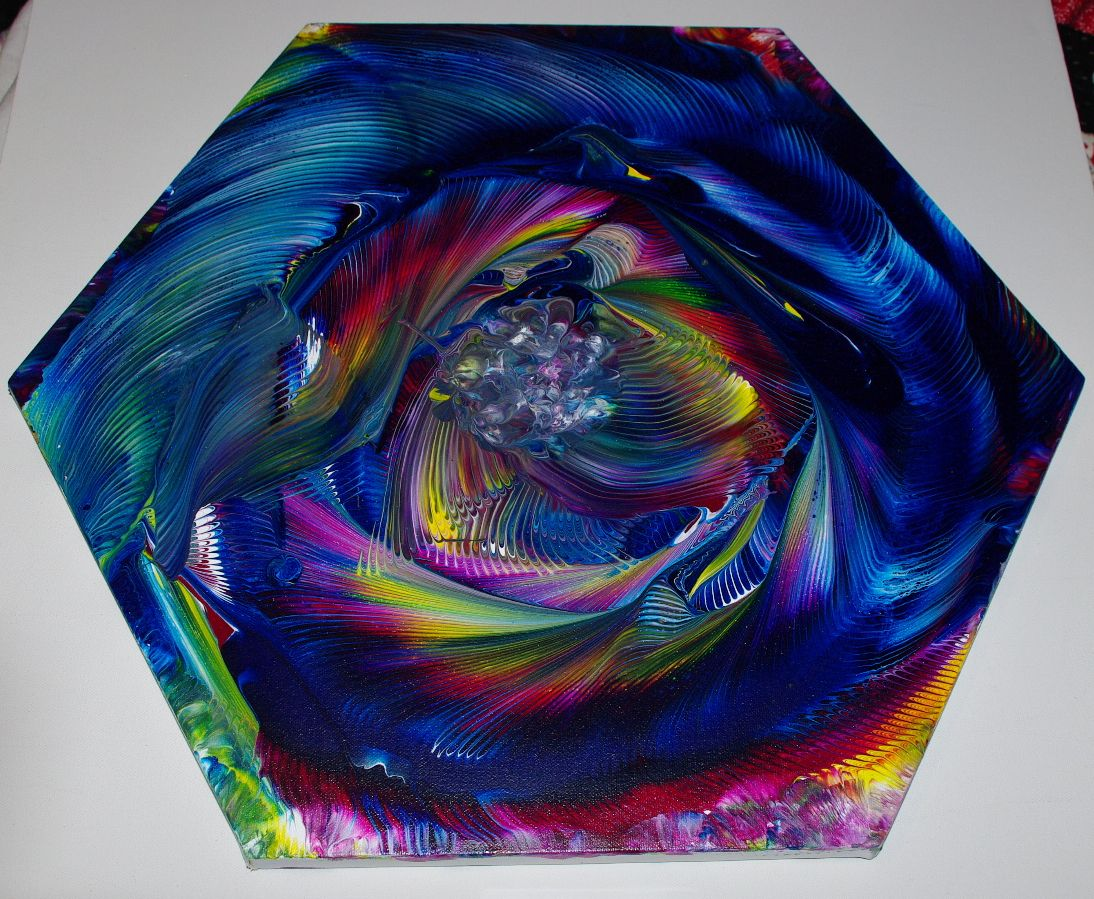Hexagon is 17" x 21"